# Homework 1 is due Homework 2 coming soon

• Slides: 33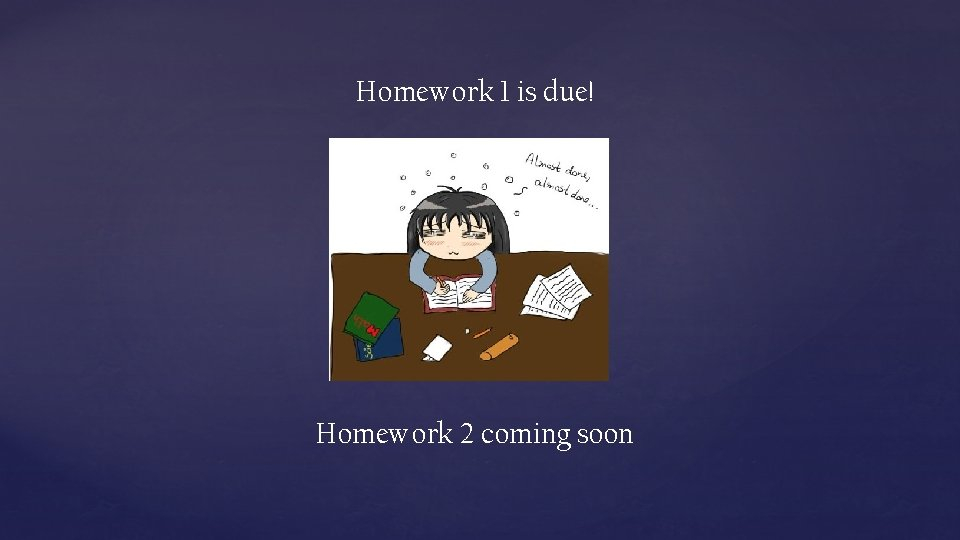Homework 1 is due! Homework 2 coming soonCS 154, Lecture 5: The Myhill-Nerode Theorem, On Learning DFAs Streaming Algorithms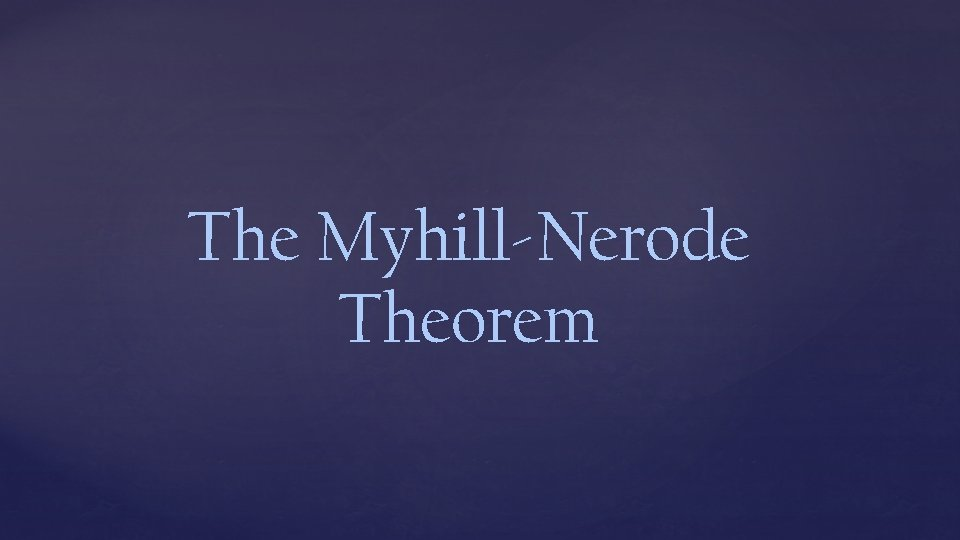The Myhill-Nerode Theorem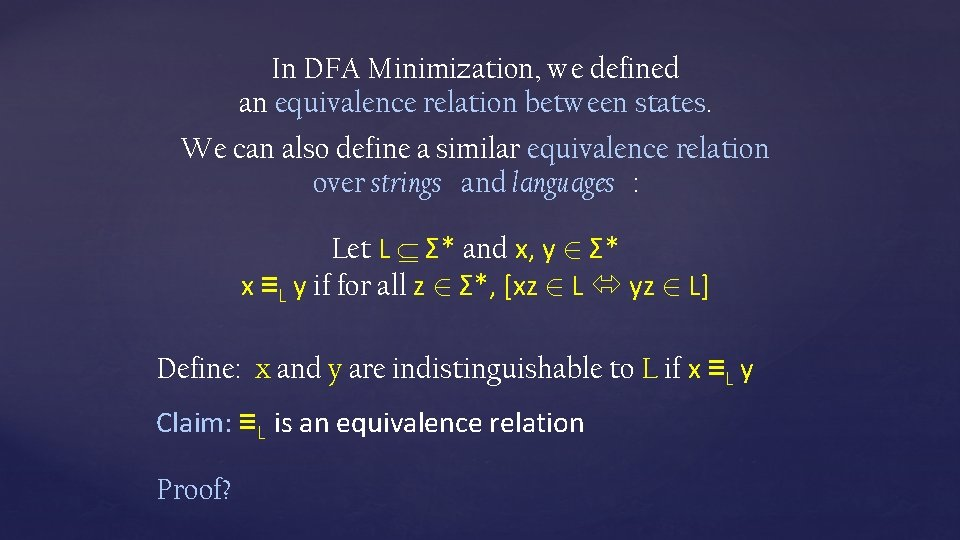In DFA Minimization, we defined an equivalence relation between states. We can also define a similar equivalence relation over strings and languages : Let L Σ* and x, y 2 Σ* x ≡L y if for all z 2 Σ*, [xz 2 L yz 2 L] Define: x and y are indistinguishable to L if x ≡L y Claim: ≡L is an equivalence relation Proof?Let L Σ* and x, y 2 Σ* x ≡L y if for all z 2 Σ*, [xz 2 L yz 2 L] The Myhill-Nerode Theorem: A language L is regular if and only if the number of equivalence classes of ≡L is finite. Proof (⇒) Let M = (Q, Σ, , q 0, F) be a min DFA for L. Define the relation: x ~M y if (q 0, x) = (q 0, y) Claim: ~M is an equivalence relation with |Q| classes Claim: If x ~M y then x ≡L y Proof: x ~M y implies for all z Σ*, xz and yz reach the same state of M. So xz L yz L, and x ≡L y Corollary: Number of equivalence classes of ≡L is at most the number of equivalence classes of ~M (which is |Q|)Let L Σ* and x, y 2 Σ* x ≡L y if for all z 2 Σ*, [xz 2 L yz 2 L] (⇐) If the number of equivalence classes of ≡L is k then there is a DFA for L with k states Idea: Build a DFA using equivalence classes of ≡L Define a DFA M where Q is the set of equivalence classes of ≡L q 0 = [ε] = {y | y ≡L ε} ([x], ) = [x ] F = {[x] | x L} Claim: M accepts x if and only if x L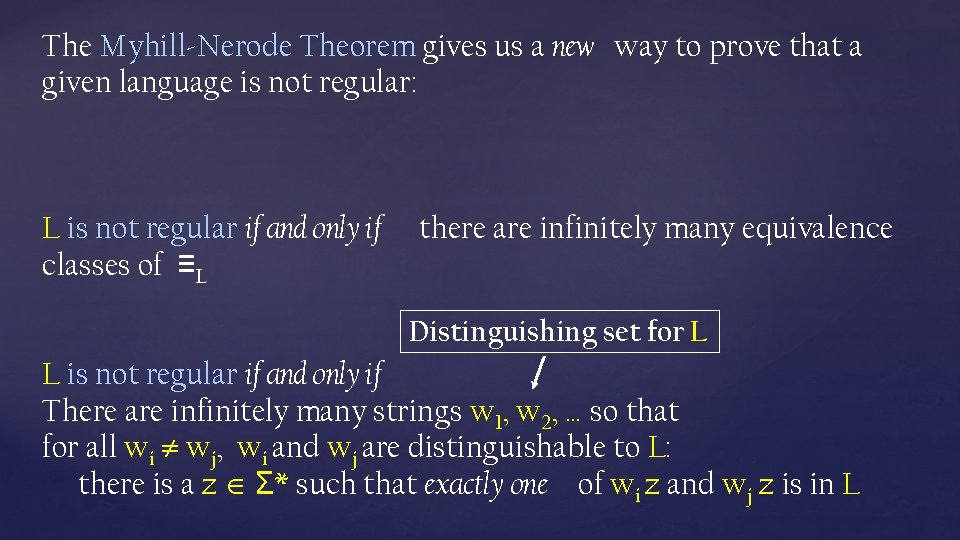The Myhill-Nerode Theorem gives us a new way to prove that a given language is not regular: L is not regular if and only if classes of ≡L there are infinitely many equivalence Distinguishing set for L L is not regular if and only if There are infinitely many strings w 1, w 2, … so that for all wi wj, wi and wj are distinguishable to L: there is a z Σ* such that exactly one of wi z and wj z is in L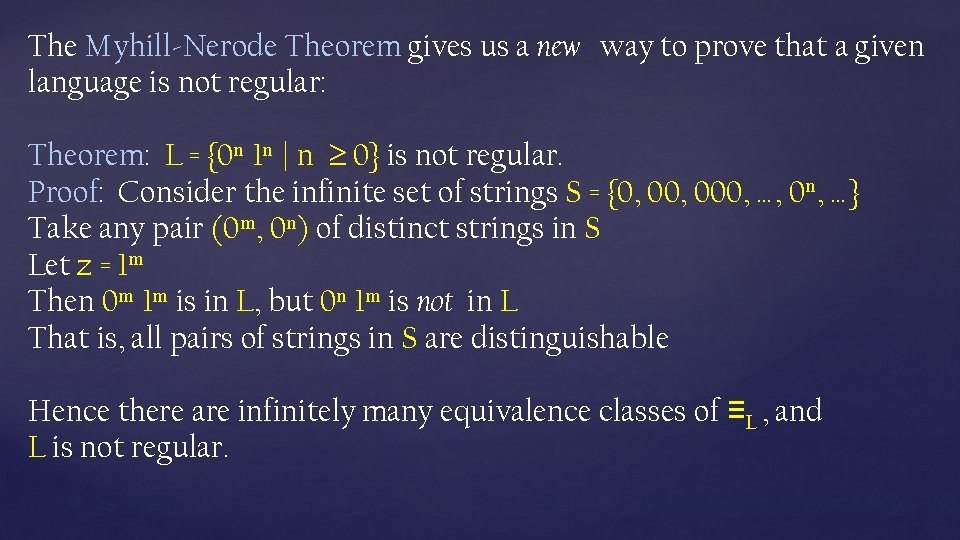The Myhill-Nerode Theorem gives us a new way to prove that a given language is not regular: Theorem: L = {0 n 1 n | n 0} is not regular. Proof: Consider the infinite set of strings S = {0, 000, …, 0 n, …} Take any pair (0 m, 0 n) of distinct strings in S Let z = 1 m Then 0 m 1 m is in L, but 0 n 1 m is not in L That is, all pairs of strings in S are distinguishable Hence there are infinitely many equivalence classes of ≡L , and L is not regular.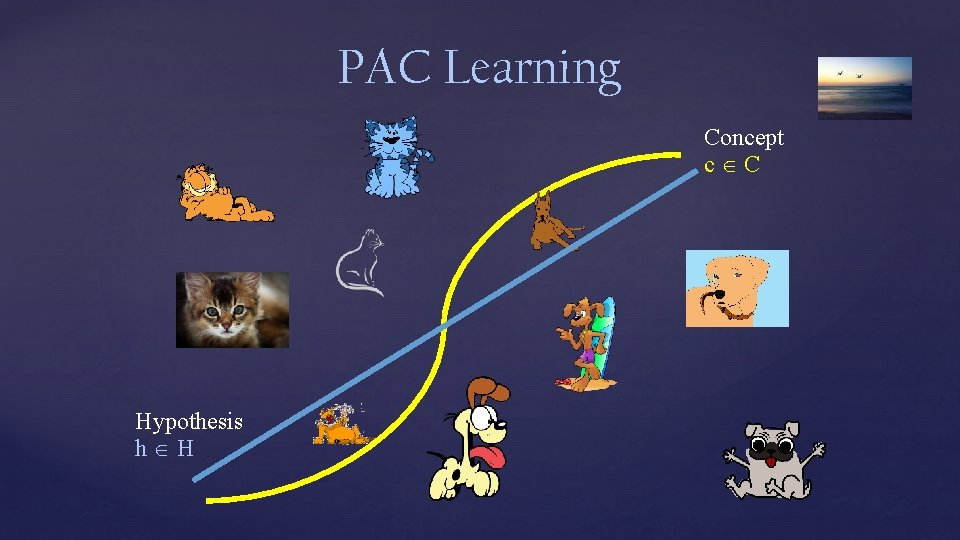PAC Learning Concept c C Hypothesis h H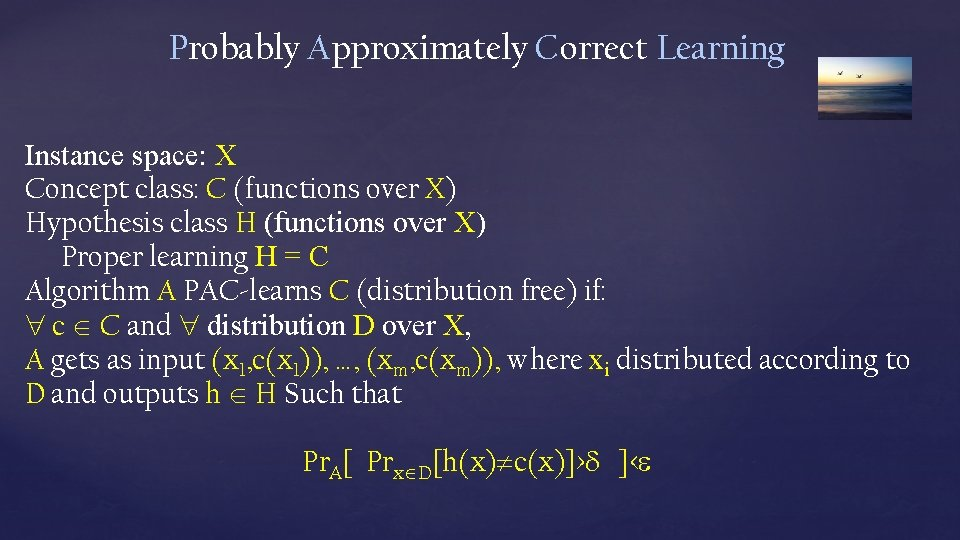Probably Approximately Correct Learning Instance space: X Concept class: C (functions over X) Hypothesis class H (functions over X) Proper learning H = C Algorithm A PAC-learns C (distribution free) if: c C and distribution D over X, A gets as input (x 1, c(x 1)), …, (xm, c(xm)), where xi distributed according to D and outputs h H Such that Pr. A[ Prx D[h(x) c(x)]> ]<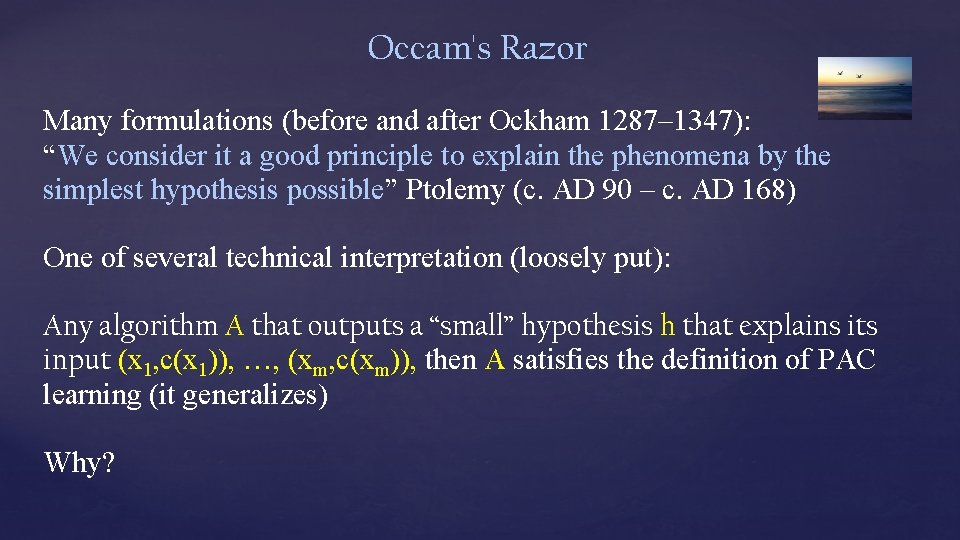Occam's Razor Many formulations (before and after Ockham 1287– 1347): “We consider it a good principle to explain the phenomena by the simplest hypothesis possible” Ptolemy (c. AD 90 – c. AD 168) One of several technical interpretation (loosely put): Any algorithm A that outputs a “small” hypothesis h that explains its input (x 1, c(x 1)), …, (xm, c(xm)), then A satisfies the definition of PAC learning (it generalizes) Why?PAC-Learning DFA Given a regular language L, and given examples w 1 … wm (positive and negative), learn a small DFA that is consistent with L on these inputs. One approach: enumerate all DFAs (from smallest) till you find one that is consistent with input. Exponential in size of DFA. Can we do better?PAC-Learning DFA Given a regular language L, and given examples w 1 … wm (positive and negative), learn a DFA that is consistent with L on these inputs. Define L? to be the corresponding “partially defined language” such that either w L? or we don’t know. L? distinguishes x and y if there exist z such that either xz 2 L? and yz L? or vise versa. Claim: if x ≡L y then x and y cannot be distinguished by L? Use similar approach to the proof of Myhill-Nerode ? ?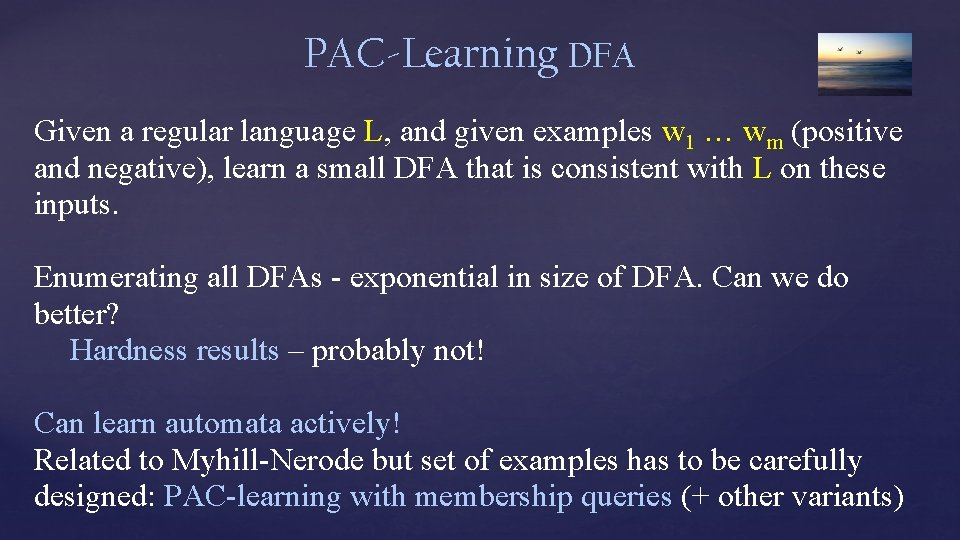PAC-Learning DFA Given a regular language L, and given examples w 1 … wm (positive and negative), learn a small DFA that is consistent with L on these inputs. Enumerating all DFAs - exponential in size of DFA. Can we do better? Hardness results – probably not! Can learn automata actively! Related to Myhill-Nerode but set of examples has to be carefully designed: PAC-learning with membership queries (+ other variants)Streaming Algorithms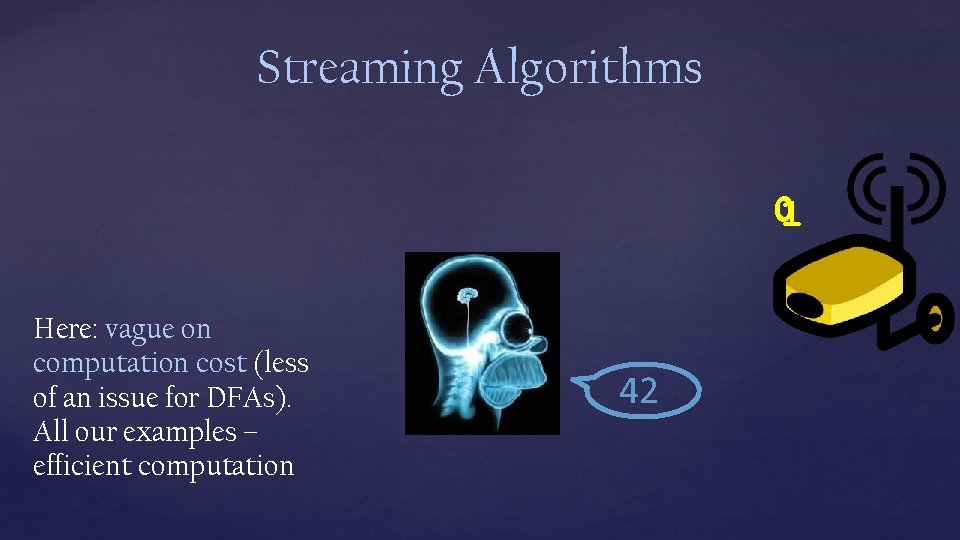Streaming Algorithms 01 Here: vague on computation cost (less of an issue for DFAs). All our examples – efficient computation 42L = {x | x has more 1’s than 0’s} Initialize: C : = 0 and B : = 0 When the next symbol is read, If (C = 0) then B : = , C : = 1 If (C 0) and (B = ) then C : = C + 1 If (C 0) and (B ) then C : = C – 1 When the stream stops, accept if B=1 and C > 0, else reject B = the majority bit C = how many more times that B appears On all strings of length n, the algorithm uses (1+log 2 n) bits of space (to store B and C )Streaming Algorithms 01011101 Streaming algorithms differ from DFAs in several significant ways: 1. Streaming algorithms can output more than one bit Can recognize non-regular languages 2. The “memory” or “space” of a streaming algorithm can (slowly) increase as it reads longer strings 3. Sometimes allow making multiple passes over the data; Could be randomized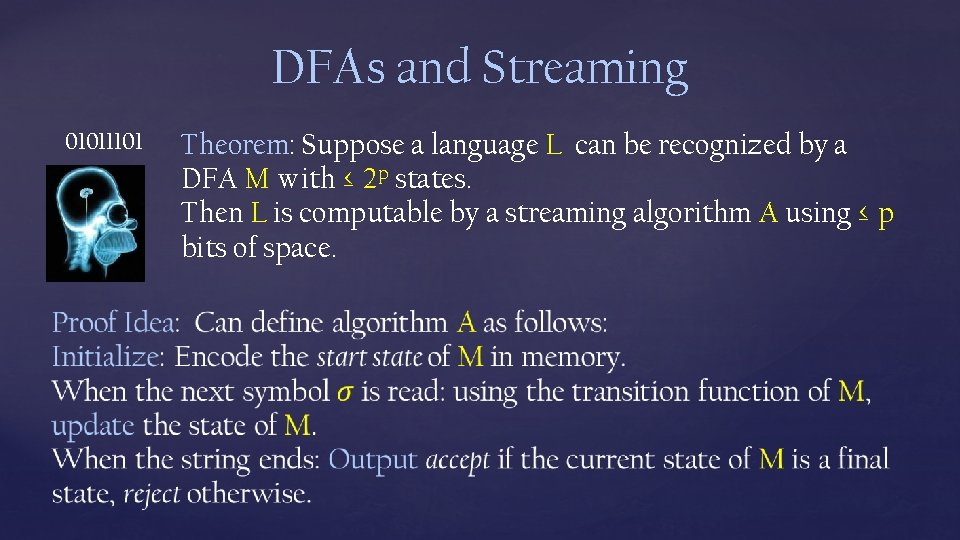DFAs and Streaming 01011101 Theorem: Suppose a language L can be recognized by a DFA M with ≤ 2 p states. Then L is computable by a streaming algorithm A using ≤ p bits of space.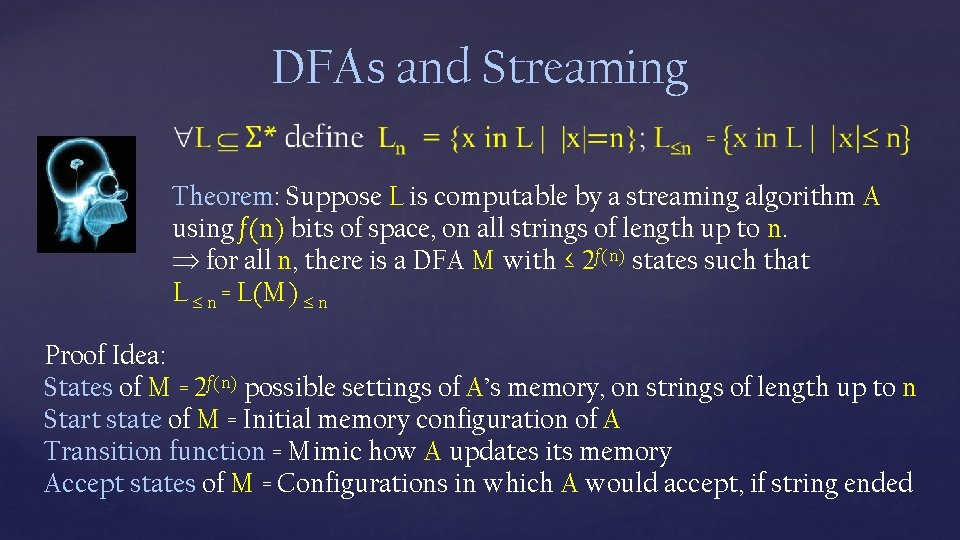DFAs and Streaming Theorem: Suppose L is computable by a streaming algorithm A using f(n) bits of space, on all strings of length up to n. for all n, there is a DFA M with ≤ 2 f(n) states such that L n = L(M) n Proof Idea: States of M = 2 f(n) possible settings of A’s memory, on strings of length up to n Start state of M = Initial memory configuration of A Transition function = Mimic how A updates its memory Accept states of M = Configurations in which A would accept, if string ended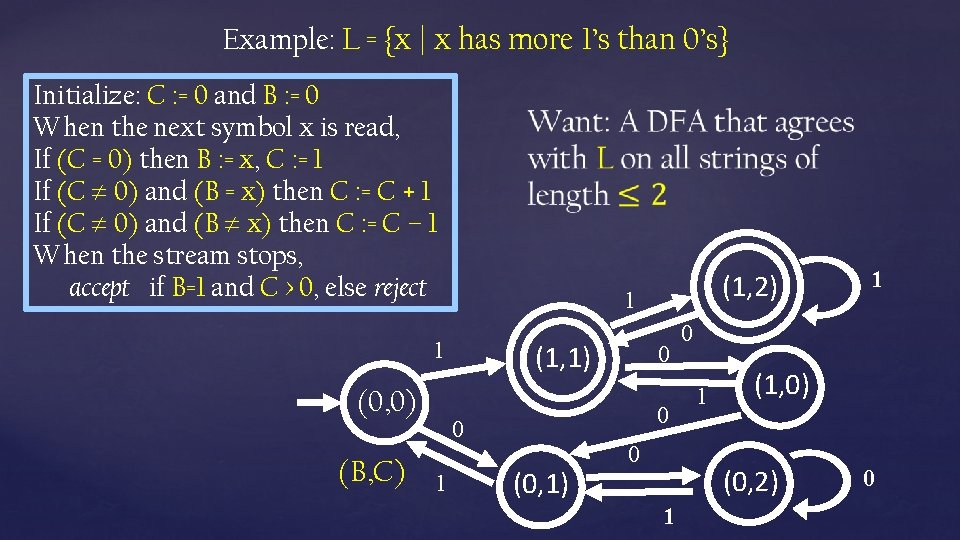Example: L = {x | x has more 1’s than 0’s} Initialize: C : = 0 and B : = 0 When the next symbol x is read, If (C = 0) then B : = x, C : = 1 If (C 0) and (B = x) then C : = C + 1 If (C 0) and (B x) then C : = C – 1 When the stream stops, accept if B=1 and C > 0, else reject 1 1 (0, 0) (B, C) (1, 1) 0 0 0 1 (1, 2) (0, 1) 0 0 1 (1, 0) (0, 2) 1 1 0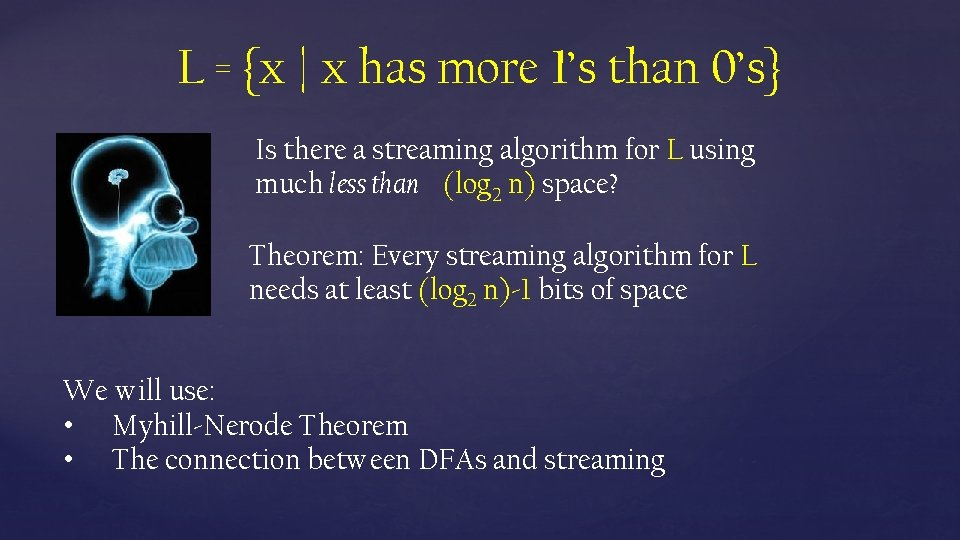L = {x | x has more 1’s than 0’s} Is there a streaming algorithm for L using much less than (log 2 n) space? Theorem: Every streaming algorithm for L needs at least (log 2 n)-1 bits of space We will use: • Myhill-Nerode Theorem • The connection between DFAs and streaming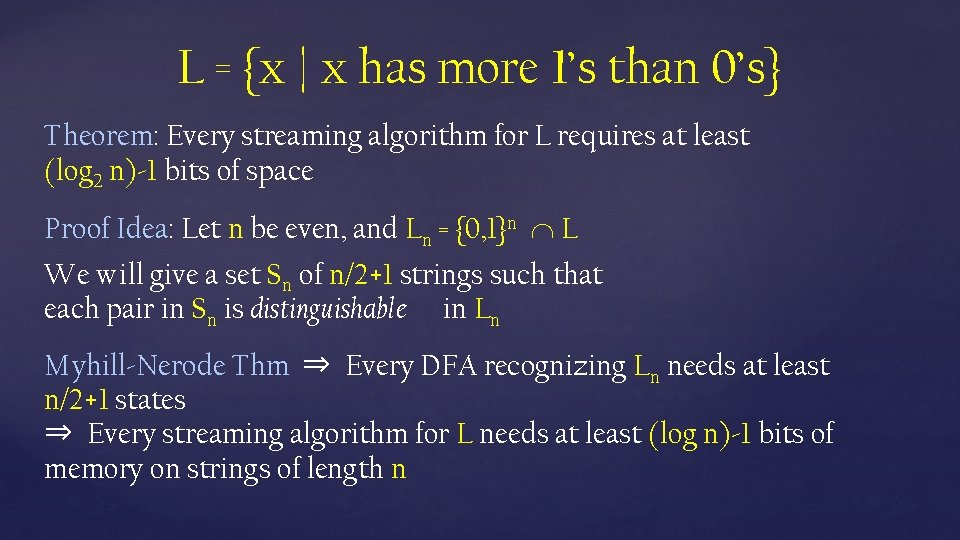L = {x | x has more 1’s than 0’s} Theorem: Every streaming algorithm for L requires at least (log 2 n)-1 bits of space Proof Idea: Let n be even, and Ln = {0, 1}n L We will give a set Sn of n/2+1 strings such that each pair in Sn is distinguishable in Ln Myhill-Nerode Thm ⇒ Every DFA recognizing Ln needs at least n/2+1 states ⇒ Every streaming algorithm for L needs at least (log n)-1 bits of memory on strings of length nL = {x | x has more 1’s than 0’s} Theorem: Every streaming algorithm for L requires at least (log 2 n)-1 bits of space Suppose we partition all strings into their equivalence classes under ≡Ln But the number of states in a DFA recognizing Ln is at least the number of equivalence classes under ≡Ln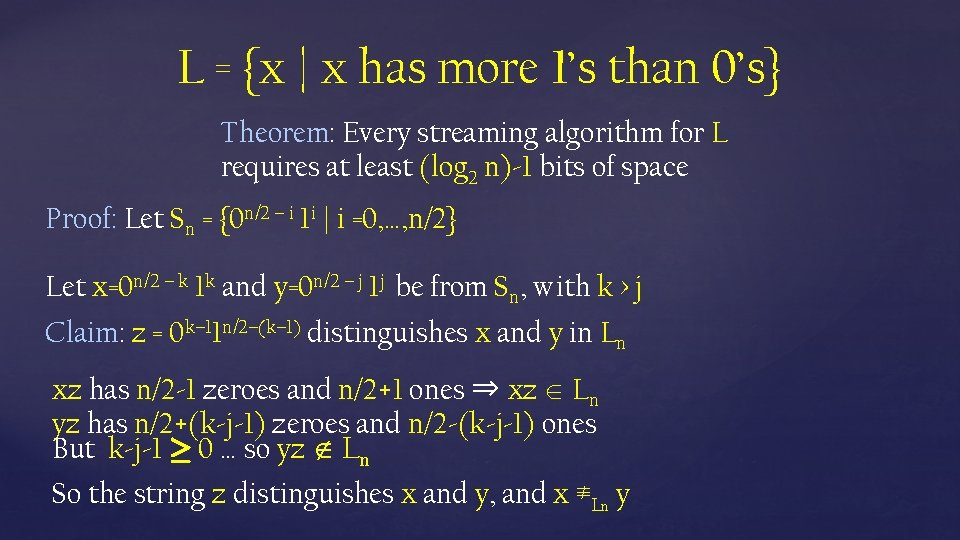L = {x | x has more 1’s than 0’s} Theorem: Every streaming algorithm for L requires at least (log 2 n)-1 bits of space Proof: Let Sn = {0 n/2 – i 1 i | i =0, …, n/2} Let x=0 n/2 – k 1 k and y=0 n/2 – j 1 j be from Sn, with k > j Claim: z = 0 k– 11 n/2–(k– 1) distinguishes x and y in Ln xz has n/2 -1 zeroes and n/2+1 ones ⇒ xz Ln yz has n/2+(k-j-1) zeroes and n/2 -(k-j-1) ones But k-j-1 ¸ 0 … so yz Ln So the string z distinguishes x and y, and x ≢Ln yL = {x | x has more 1’s than 0’s} Theorem: Every streaming algorithm for L requires at least (log 2 n)-1 bits of space Proof: All pairs of strings in Sn are distinguishable in Ln ⇒ There at least |Sn| equiv classes of ≡Ln By the Myhill-Nerode Theorem: ⇒ All DFAs recognizing Ln need ¸ |Sn| states ⇒ Every streaming algorithm for L requires at least (log 2 |Sn|) bits of space. Recall |Sn|=n/2+1 and we’re done!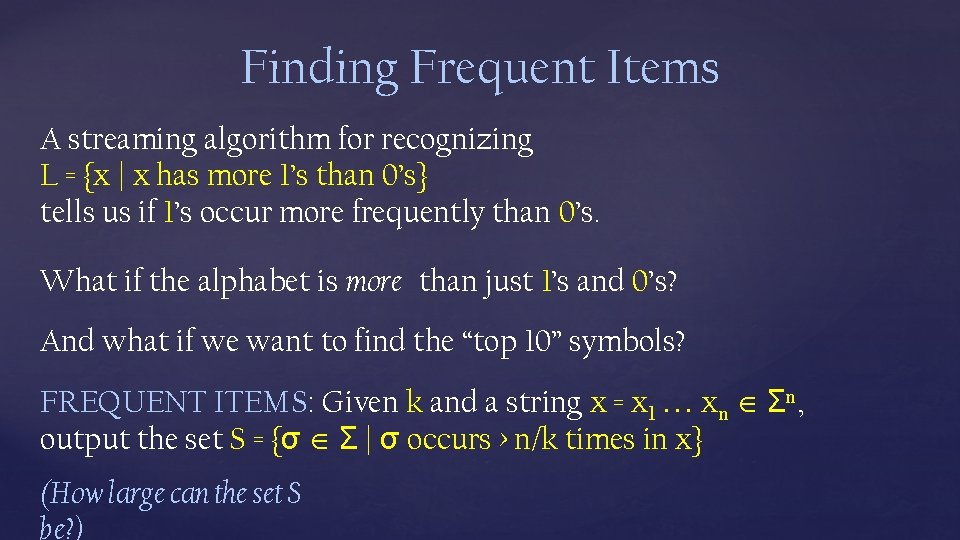Finding Frequent Items A streaming algorithm for recognizing L = {x | x has more 1’s than 0’s} tells us if 1’s occur more frequently than 0’s. What if the alphabet is more than just 1’s and 0’s? And what if we want to find the “top 10” symbols? FREQUENT ITEMS: Given k and a string x = x 1 xn Σn, output the set S = {σ Σ | σ occurs > n/k times in x} (How large can the set S be? )FREQUENT ITEMS: Given k and a string x = x 1 xn Σn, output the set S = {σ Σ | σ occurs > n/k times in x} Theorem: There is a two-pass streaming algorithm for FREQUENT ITEMS using O(k (log |Σ| + log n)) space. 1 st pass: Initialize an set T ⊆ Σ x ℕ (originally empty) Read the next symbol σ from the stream. If (σ, m) T, then T : = T – {(σ, m)} + {(σ, m+1)} Else if |T| < k-1 then T : = T + {(σ, 1)} Else for all (σ’, m’) T, T : = T – {(σ’, m’)} + {(σ’, m’-1)} If m’ = 0 then T : = T – {(σ’, m’)} Claim: T contains all σ occurring > n/k times in x 2 nd pass: Count occurrences of all σ’ appearing in T to determine those occurring > n/k times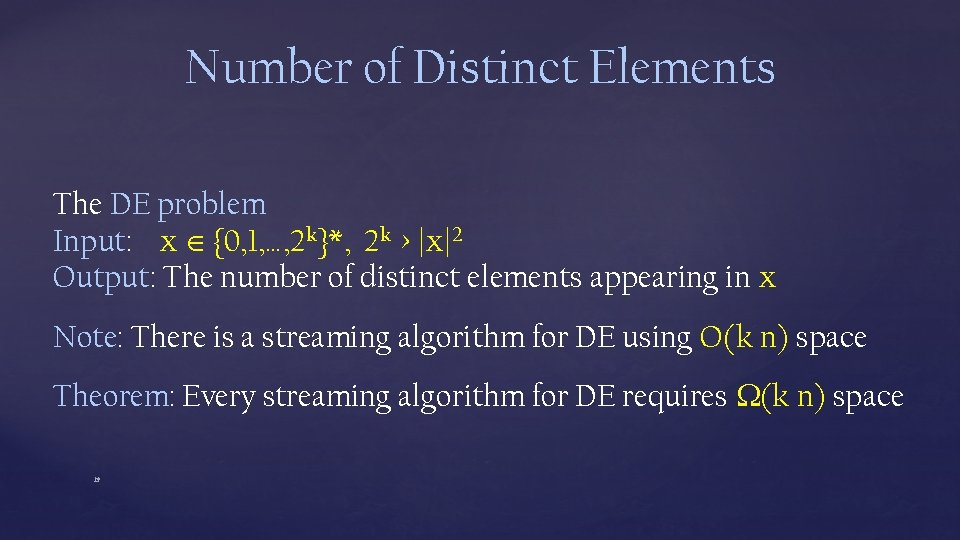Number of Distinct Elements The DE problem Input: x {0, 1, …, 2 k}*, 2 k > |x|2 Output: The number of distinct elements appearing in x Note: There is a streaming algorithm for DE using O(k n) space Theorem: Every streaming algorithm for DE requires (k n) space 29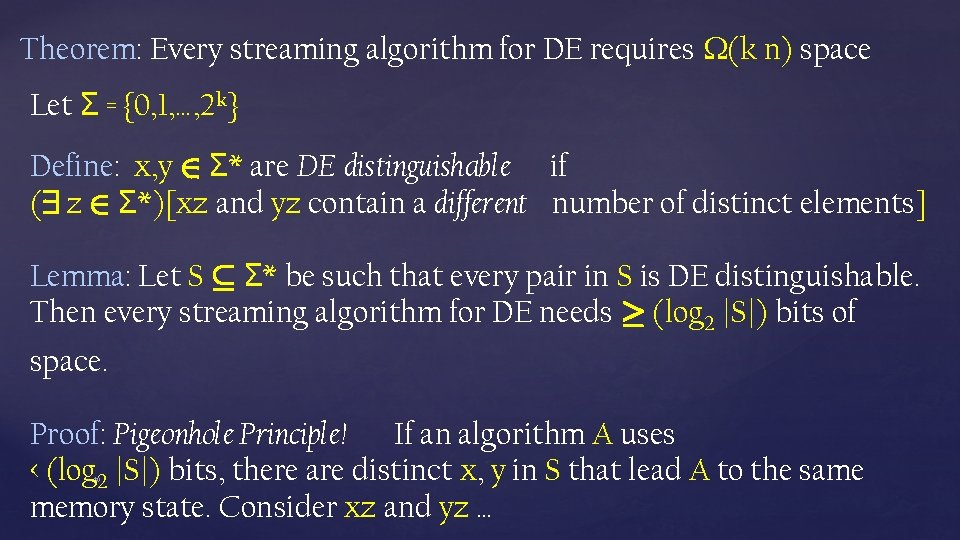Theorem: Every streaming algorithm for DE requires (k n) space Let Σ = {0, 1, …, 2 k} Define: x, y 2 Σ* are DE distinguishable if (9 z 2 Σ*)[xz and yz contain a different number of distinct elements] Lemma: Let S µ Σ* be such that every pair in S is DE distinguishable. Then every streaming algorithm for DE needs ¸ (log 2 |S|) bits of space. Proof: Pigeonhole Principle! If an algorithm A uses < (log 2 |S|) bits, there are distinct x, y in S that lead A to the same memory state. Consider xz and yz … 30Theorem: Every streaming algorithm for DE requires (k n) space Lemma: Let S µ Σ* be such that every pair in S is DE distinguishable. Then every streaming algorithm for DE needs ¸ (log 2 |S|) bits of space. Lemma: There is a DE distinguishable S of size 2 (k n) Proof: For each subset T of Σ of size n/2, define x. T to be any concatenation of the strings in T For distinct sets T and T’, x. T and x. T’ are distinguishable: x. T contains exactly n/2 distinct elements x. T’ x. T has more than n/2 distinct elements The total number of such subsets is 2 (k n), for 2 k > n 2.Randomized Algorithms Help! The DE problem Input: x {0, 1, …, 2 k}*, 2 k > |x|2 Output: The number of distinct elements appearing in x Theorem: There is a randomized streaming algorithm that can approximate DE to within 0. 1% error, using O(k + log n) space! Suppose: the elements are selected uniformly at random. What can we say about the minimal element? If we have at our disposal a random permutation h over {0, 1, …, 2 k} what can we do? Derandomizatrion: h that is efficient and have short description.Parting thoughts: Myhill-Nerode Theorem – powerful characterization Streaming – modern day incarnations of DFAs Randomness – could be a useful resource of computation Questions?